Next: Computation of coefficients Up: Definitions Previous: Interferogram   Contents

# Polynomials

A 1d-polynomial is defined as: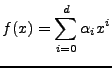(D25)

A 2d-polynomial is defined as: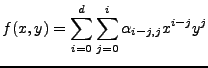(D26)

Thus the order of the coefficients (line,pixel) is independent of degree d:
d=0:(1)
d=1: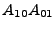(2, 3)
d=2: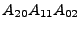(4, 5, 6)
d=3: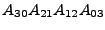(7, 8, 9, 10)

Thus the number of coefficients (unknowns in least squares estimation) equals for a 2d-polynomial of degree d: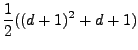(D27)

And the degree of a polynomial with N coefficients is equal to: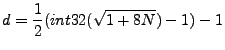(D28)

Subsections

Leijen 2009-04-14